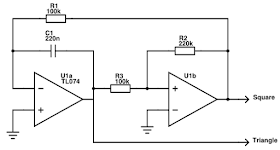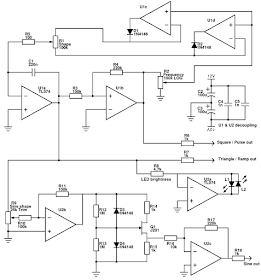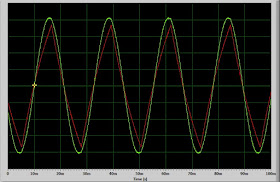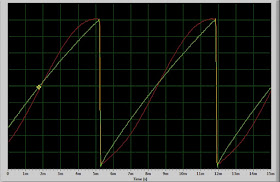## Oct 18, 2015

### Variable waveshape LFO

This is a fairly simple LFO circuit, whose wave shape can be smoothly varied between downward ramp, triangle and upward ramp. In addition, a square/pulse wave and a sine wave output are given.

### Original circuit

Below is a basic and common triangle oscillator circuit, consisting of an integrator (U1a, R1, C1) and a Schmitt trigger (U1b, R2, R3).Triangle oscillator

It works as follows:
• Assume U1b's output is high (the positive rail, Vsupply). This makes a current flow through R1 and C1. Charge build-up in C1 means output of U1a must decrease linearly in time.
• When the output of U1a goes below about -Vsupply/2, the + input of U1b goes below ground and the output of U1b switches to negative (-Vsupply).
• The current reverses, and the voltage at U1b output starts to increase again until it reaches +Vsupply/2.
• U1b goes high again, and the cycle repeats.
The oscillation period is determined by R1*C1 (times some constant, see here for the formulas)

### Variable waveshape version

The basic idea is to use a different value of R1 for charging and for discharging, so that the up ramp and down ramp have different periods. One can use two resistors (or pots), and diodes to force the current for each ramp through the right resistor. However, it would be nicer to have independent control of shape and frequency. Here is the solution I ended up with (note: it turns out that Ken Stone uses the same idea here)Variable waveshape LFO core

R1 is replaced by a potentiometer (plus a small offset R5), where each half is fed by a diode. One diode conducts in the up ramp, and one in the down ramp. The total cycle time stays constant independent of shape. Frequency is controlled by R2, which reduces the output voltage of U1b and thereby the charging/discharging current.

The diodes are actually implemented as precision rectifiers: U1c and U1d compensate the 0.6 V voltage drop of the diodes. This gives the oscillator a very wide frequency range: with the component values as shown, it goes up to about 40 Hz, and at the slowest setting one oscillation takes 10s of seconds. In addition, the opamps buffer the output of R2, which keeps sharp edges on the ramp setting even at low frequency.

R5 is intended to limit the current a bit more than the opamp drive capability, to reduce frequency change when setting R1 to one of its extreme settings. But the value of R5 is a compromise, and can be adjusted or even omitted.

The frequency range can be changed as desired by changing the value of C1 and/or R1.

### Sine-wave converter

I wanted to try out a classic and very simple triangle-to-sine conversion circuit based on a JFET transistor, and it worked so well I kept it in this LFO. More info and references can be found on this page. The basic circuit has a nice symmetry:Triangle-to-sine conversion

The JFET is in this case always forward-biased, contrary to their normal usage. Depending on the polarity of the input, D3 or D4 limits the JFETs gate voltage. Saturation of the JFET then gives the triangle-to-sine conversion. The input level needs to be adjusted carefully to get the right amount of saturation, but it gives a very nice sine wave, reaching less then 1% total harmonic distortion (THD) in my circuit.

It seems almost any JFET will work to give good sine waves, but the input voltage and current levels where it works vary wildly between models (and even between each one, but usually they are tested to fall in a certain range). JFETs are not too easy to get as they are rarely used in modern electronics, which is also the biggest downside of this circuit. From the options I had, J201 seemed reasonable, with a zero-gate-voltage resistance of about 2 kOhm. This sets the scale for R14 and R15, and thereby the total voltage and current needs. The optimal input amplitude was about 1.1 V for my circuit. A positive surprise was that the J201 worked much better in this application than I had expected from simulations.

### Complete circuit

Below is the complete circuit. In addition to the parts discussed above, it contains input level scaling (R9 trimpot), input buffering (U2b) and output buffering and level restoration (U2c) for the sine wave converter. A bi-color LED (L1, L2) is added to visually show the triangle output, using U2a as bi-directional current driver.Variable waveshape LFO (for personal use only)
The triangle and sine wave have about 5 V amplitude, the square wave goes full swing of the opamp, about 10.5 V.

### ResultsThe circuit built on a small protoboard

Here is a plot of the triangle and sine wave shape (Shape pot centered) at the fastest setting. In this case, the sine wave had a THD of 0.9%. The non-linearity of the triangle wave is because I recorded the trace through a sound card (using this program).Triangle (red) and sine (green) wave

And here is a recording of the ramp wave (Shape pot maximal). The sine output also gives an interesting shape in this case.Up ramp (green) and half sinusoid (red)

### Limitations and ideas

• The edges of the ramp are fast enough for most uses, but at extremely slow settings the edges are a few ms long. Enough to hear for example in a frequency ramp. This could perhaps be improved by using opamps with larger drive capability for U1c/U1d.
• Frequency changes a bit (up to about 10%) with changing the shape. The reason might be that the supply voltages are not exactly symmetric in my system.
• Frequency will change directly with supply voltage in general. If you care about this a lot, it might be easier to go for ramp/saw style oscillators that use only a single reference voltage, and use a precision voltage reference to define it.